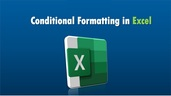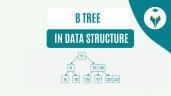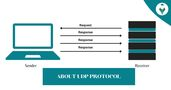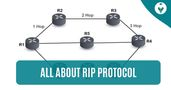Absolute Reference in Excel – Shiksha Online

# Absolute Reference in Excel – Shiksha Online

clickHere
Updated on Mar 3, 2023 12:58 IST

The article covers what is absolute referencing in Excel, how to create an absolute reference, and examples of absolute referencing.

You aim to achieve relative referencing when you copy a formula in the cells, making it the default behavior of Excel. However, the objective might be to apply absolute references and not relative references. You will understand this by the example that we have mentioned below.

The absolute reference makes a cell reference fixed to an absolute, or we can say a defined cell address. It always refers to the same cell and therefore does not allow it to be modified even when copying the formula.

Content

The importance of absolute references is that they remain fixed, which is very useful when working with Excel functions or formulas.

Must Read – Difference Between Formula and Function in Excel

## How to Create an Absolute Reference in Excel

The absolute reference is created by placing the dollar sign (\$) before the column letter and before the row number of the cell we want to set, for example: =\$F\$2Most Popular and Powerful Formulas in Excel
The article discusses the most popular formulas in excel through practical examples of the use of formulas for Excel.Average Function in Excel – Formula and Examples
The AVERAGE function is a popular Excel function used for various purposes, such as calculating average sales, average student scores, average monthly expenses, etc. It provides a quick and efficient...read moreTop MS Excel Interview Questions
Master your Excel interview with confidence using our curated list of top questions. From basic functions to advanced data analysis, boost your Excel proficiency and shine in any data-driven role.

### Absolute Reference Shortcut

To make absolute reference, we have several ways. One of them is by manually typing the dollar sign (\$), or the other is by pressing the F4 key on our keyboard. The latter would be much faster and is recommended to avoid any manual error. For this, you just need to select cell F2 and press F4, the result will be \$F\$2.

## Example 1 – Absolute Referencing

To give you a more precise explanation of how to create an absolute reference in Excel, we will continue with the example. We will convert the value of the products in Dollars to Rupees. For this, we will put the exchange value or exchange rate in another cell, F2.

We will put the change value in cell F2, and place that absolute reference so that when copying, it does not alter the information. If we were to do this without making the cell fixed, we would have the following result.

With this, we can validate that the information is not copied correctly. So that our example can have the expected result, we only have to fix the cell. This is where absolute references in Excel are crucial.

### Make an Absolute Reference

Continuing with our example, we are going to locate ourselves in cell C3, and we are going to write our formula, which in our case, is a simple formula. We would have the following =B3*F2. Since we have not yet fixed cell F2, we won’t be able to make an absolute reference. We will use the formula

=B3*\$F\$2.

It is also important to note that we are working with a relative reference that is B3, and at the same time, we have created an absolute reference. Now, we would only have to copy or drag so that the formula applies to other cells.

You can see that when we copied the absolute references formula in the rest of the cells, Excel made the correct calculation and you get the desired results for all the entries.What is Conditional Formatting in Excel?
Learn what is Conditional Formatting in Excel and explore different presets of Conditional Formatting to analyze and present your data in the most effective and powerful way.Introduction to MS Excel – A Beginner’s Guide
Let’s gets started with MS Excel, the most useful tool to crunch numbers and derive information for data analysts and scientists.How to Create MIS Reports in Excel?
MIS reports are crucial to measure the business performance and contribute towards meeting the overall business goals. In this article, you will learn easy steps to create an MIS report...read more

## Example 2 – Absolute Referencing

Absolute references are very popular in business data analysis, especially when dealing with fixed values ​​such as VAT.

In this example, we aim to get a profit for a company. We have the sales data and monthly production costs. In addition, we also have fixed expenses per month.

In column B, we have the sales data and in column C, monthly costs. We will calculate the profit in column D. In addition, cell A10 contains the fixed cost that is repeated every month. The formula would be as follows:

#### Absolute Reference formula

Sales – (Production costs – Fixed cost)

In Excel, it would translate as –

=B2-(C2+\$A\$10)

The total formula combines relative Excel references and an absolute Excel reference.

When we extend the formula to the rest of the cells in the Profits column, we see how the result is applied to each cell.

The relative references of the sale and the cost of production are adapted to each row, while the absolute reference of the fixed cost of the month remains fixed.

Thus, in cell D2 the formula is =B2-(C2+\$A\$10),

In cell D3, the formula is =B3-(C3+\$A\$10), until cell D7 =B7-(C7+\$A\$10).

As we can see, absolute references are used so that the content of a specific cell is not affected if we copy and paste the formula to another cell in Excel.

This is how we create absolute references in Excel. We hope that this article helped you to understand what is absolute reference in Excel.

## FAQs

What is absolute reference?

A reference is absolute when Excel cannot adjust it to fit the formula as it changes rows or columns. Absolute references remain constant no matter where the formula is copied and defined using the \$ symbol.

What is the importance of absolute references in Excel?

The importance of absolute references is that they remain fixed, which is very useful when working with Excel functions or formulas.

What is absolute and relative reference in Excel?

There are two types of references: relative and absolute. Relative references change when they are copied from one cell to another. Absolute references, however, remain constant no matter what cell they are copied to.

How to make an absolute reference in Excel without F4?

Excel offers us the possibility of fixing cells: To work with absolute references, it must be specified by writing the \$ sign in before the column letter and the row number.

What is Relative reference in Excel?

Relative references refer to the location of the cell, that is, to the row and column where the cell that contains a value or a formula is located; when we take that same cell to move it to another cell, worth the redundancy, this will do reference to new cells based on their location.

clickHere

Rashmi is a postgraduate in Biotechnology with a flair for research-oriented work and has an experience of over 13 years in content creation and social media handling. She has a diversified writing portfolio and aim... Read Full Bio

## Trending Management CoursesExcel for Beginners
Great Learning4.6Microsoft Excel - Excel from Beginner to Advanced
UDEMY4.7Introduction to MS Excel
Simplilearn4.6Create Charts and Dashboards Using Microsoft Excel
Coursera4.0Starts todayUsing Basic Formulas and Functions in Microsoft ExcelUsing Basic Formulas and Functions in Microsoft Ex...
Coursera5.0Starts today

## Top Picks & New Arrivals